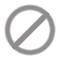##Sales Forecasting Model

The Sales Forecasting model developed by Cappius uses a user defined window to predict future value of a time series by using Linear regression. The model that could be used are Neural networks or SVMs. The model performance is also evaluated by performing Residual analysis.

Technical Description of the Model:

Data is loaded and the date column is converted to date format using ‘Nominal to Date’ operator. The date column is extended up to the date of horizon period. This is done by extracting last date from the given date column as ‘macro’ and iterating over it for a period of horizon provided. Each iteration increments the date by days, weeks, months and year (here we increment by weeks). This gives the forecast period dates which would be used in visualizing the forecast values.

‘Predict Series’ operator is used for some basic series prediction operations. The given series must be univariate and must be encoded by examples, i.e. each point of time is encoded by the values in one single example. The values which should be predicted must be defined by the label attribute. The operator creates time windows and learns a model from these windows to predict the value of the label column after a certain amount of values (horizon). Windowing width value is changed and the model is built to get the predicted value.

Model with least error is usually preferred. After predicting a value, the window is moved with step size 1 and the next value is predicted. All predictions are kept and can be compared afterwards to the actual values in a series plot or with a performance evaluation operator.

Once forecast is done, residual analysis is done to check for:

• Near zero mean of residuals
• Normal distribution of errors
• Constant variance of errors
• LJung-Box test to check for uncorrelated errors

 Version File size Downloads 0 (0 Today)Vendor Anblicks Category Machine Learning Released Last Update License Product web site www.cappius.com Rating(0)